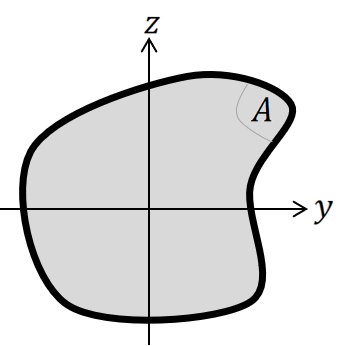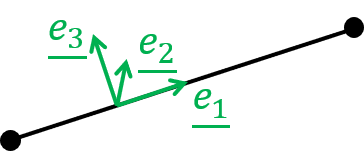user_manual:sesamx:functions_description:create_properties

# 3.5.10. SET-PROPERTIES function

optional

This function is used to define the element properties and describes how the elements will behave. There are 5 types of properties that you can define:

• the standard truss property,
• the Timoshenko beam property,
• the MITC shell property,
• the isoparametric solid property,
• and the MITC solid property.

While defining a property, you need to provide the characteristics of the property as well as the element selection on which this property applies. This page explains how to properly use each property type.

Name Data Type Data number Optional Default value Physical quantity
MODEL STRING 1 NO
Name of the model on which the properties are defined.

optional

This branch is used to define a truss property. It is compatible with the LN2 element shape only. This property is used to model slender structures subjected to an axial load.This element uses the 3 translational degrees of freedom at the nodes, and brings stiffness only along the line axis. The only relevant geometric parameter is the cross-section area.

The strain and stress states in the element are assumed to be:

$$\lbrack \varepsilon\rbrack = \begin{bmatrix} \varepsilon_{11} \\ \varepsilon_{22} \\ \varepsilon_{33} \\ 0 \\ 0 \\ 0 \end{bmatrix} \lbrack \sigma \rbrack = \begin{bmatrix} \sigma_{11} \\ 0 \\ 0 \\ 0 \\ 0 \\ 0 \end{bmatrix}$$

If you are looking for more detailed explanations, you can refer to our blog articles about the truss element.

#### Keywords

Name Data Type Data number Optional Default value Physical quantity
NAME STRING 1 NO
Name to give to the property.
AREA FLOAT 1 NO AREA
Area of the cross-section.
MATERIAL STRING 1 NO
Material name applied to this truss.
ON-ELEMENTS-FROM STRING 1 NO
Name of the selection on which to apply the property.

optional

This branch is used to define a Timoshenko beam property. It is compatible with the LN2 element shape only. This property is used to model slender structures subjected to an arbitrary loading (force and moment). The Timoshenko beam element enables shear deformation. This element is considered accurate for cross-section typical dimension less than $\cfrac{1}{8}$ of the beam length.This element uses the 3 translational and the 3 rotational degrees of freedom at the nodes, and brings stiffness in all the directions. There are multiple assumptions made by this element. They are described in the following.

1) Bending is supposed uncoupled from shearing. Therefore, the beam neutral axis must be the same as the beam shear axis.

2) The relevant geometric parameters of the beam are:

• the area of the cross-section
• the area moments of inertia of the cross-section:

$$I_y = \iint_A z^2 dydz$$ $$I_z = \iint_A y^2 dydz$$

• the product moment of inertia of the cross section:

$$I_{yz} = \iint_A yz dydz$$

• the polar moment of inertia:

$$I_{x} = \iint_A (y^2 + z^2) dydz$$

3) Furthermore, this element makes the assumption that the beam cross-section principal axes are along the $y$ and $z$ axis. Which translates to: $$I_{yz} = 0$$4) This element does not take into account the warping effect. To approximately account for this effect, the cross-section polar moment of inertia can be replaced by the torsion constant.

5) The cross-section inertia must be provided relative to the neutral axis. And the element nodes must be located on the neutral axis (no offset is considered).

6) The strain and stress states in the element are assumed to be:

$$\lbrack \varepsilon\rbrack = \begin{bmatrix} \varepsilon_{11} \\ \varepsilon_{22} \\ \varepsilon_{33} \\ \gamma_{12} \\ 0 \\ \gamma_{13} \end{bmatrix} \lbrack \sigma \rbrack = \begin{bmatrix} \sigma_{11} \\ 0 \\ 0 \\ \sigma_{12} \\ 0 \\ \sigma_{13} \end{bmatrix}$$

Besides, SesamX lets you choose the shear correction factor introduced in the Timoshenko beam theory.

7) To orientate the beam cross-section, you have to provide a basis object while defining the beam property. Let's suppose that this basis object defines 3 orthonormal vectors $(\underline{u_1}, \underline{u_2}, \underline{u_3})$. Then, the local axis system $(\underline{e_1}, \underline{e_2}, \underline{e_3})$ is defined on the element such as:

• $\underline{e_1} = sign(\underline{N_1N_2} \cdot \underline{u_1}) \frac {\underline{N_1N_2}} {\|\underline{N_1N_2}\|}$, where $\|\underline{N_1N_2}\|$ is the vector going from the first node of the element to its second node.
• $\underline{e_2} = \underline{u_2} - (\underline{u_2} \cdot \underline{e_1}) \underline{u_1}$ and then $\underline{u_2} = \frac{\underline{u_2}}{\|\underline{u_2}\|}$
• $\underline{e_3} = \underline{e_1} \times \underline{e_2}$If you are looking for more detailed explanations, you can refer to our blog articles about the beam element.

#### Keywords

Name Data Type Data number Optional Default value Physical quantity
NAME STRING 1 NO
Name to give to the property.
AREA FLOAT 1 NO AREA
Area of the cross-section.
J FLOAT 1 NO G-INTERTIA
Torsion constant of the cross-section.
I22 FLOAT 1 NO G-INTERTIA
Area moment of inertia of the cross-section along the $y$ axis.
I33 FLOAT 1 NO G-INTERTIA
Area moment of inertia of the cross-section along the $z$ axis.
K2 FLOAT 1 YES 1.
Shear correction factor along the $y$ axis.
K3 FLOAT 1 YES 1.
Shear correction factor along the $z$ axis.
BASIS STRING 1 NO
Name of the basis used to orient the cross-section.
MATERIAL STRING 1 NO
Material name applied to this truss.
ON-ELEMENTS-FROM STRING 1 NO
Name of the selection on which to apply the property.

optional

This branch is used to define a MITC shell property. It is compatible with the TR3 and QD4 element shapes only. This property is used to analyze thin structures subjected to an arbitrary loading (force and moment). The structure can be flat or curved and it should have one dimension smaller than the other 2.This element uses the 3 translational and the 3 rotational degrees of freedom at the nodes, and brings stiffness in all the directions except for the rotation normal to the shell surface. The only relevant geometric parameter is the shell thickness.

The strain and stress states in the element are assumed to be:

$$\lbrack \varepsilon\rbrack = \begin{bmatrix} \varepsilon_{11} \\ \varepsilon_{22} \\ \varepsilon_{33} \\ \gamma_{12} \\ \gamma_{23} \\ \gamma_{13} \end{bmatrix} \lbrack \sigma \rbrack = \begin{bmatrix} \sigma_{11} \\ \sigma_{22} \\ 0 \\ \sigma_{12} \\ \sigma_{23} \\ \sigma_{13} \end{bmatrix}$$

If you are looking for more detailed explanations, you can refer to our blog articles about the QD4 shell element and the TR3 shell element.

#### Keywords

Name Data Type Data number Optional Default value Physical quantity
NAME STRING 1 NO
Name to give to the property.
TH FLOAT 1 NO THICKNESS
Thickness of the shell.
MATERIAL STRING 1 NO
Material name applied to this truss.
ON-ELEMENTS-FROM STRING 1 NO
Name of the selection on which to apply the property.

optional

This branch is used to define an isoparametric solid property. It is compatible with the TE4, TE10, and WE6 element shapes. This property is used to analyze arbitrary structures, and it does not embed particular assumption about the element behavior.This element uses the 3 translational degrees of freedom at the nodes, and brings stiffness in all the directions. The strain and stress states in the element are:

$$\lbrack \varepsilon\rbrack = \begin{bmatrix} \varepsilon_{11} \\ \varepsilon_{22} \\ \varepsilon_{33} \\ \gamma_{12} \\ \gamma_{23} \\ \gamma_{13} \end{bmatrix} \lbrack \sigma \rbrack = \begin{bmatrix} \sigma_{11} \\ \sigma_{22} \\ \sigma_{33} \\ \sigma_{12} \\ \sigma_{23} \\ \sigma_{13} \end{bmatrix}$$

#### Keywords

Name Data Type Data number Optional Default value Physical quantity
NAME STRING 1 NO
Name to give to the property.
MATERIAL STRING 1 NO
Material name applied to this truss.
ON-ELEMENTS-FROM STRING 1 NO
Name of the selection on which to apply the property.

optional

This branch is used to define a MITC solid property. It is compatible with the HE8 element shape only. This property is used to analyze arbitrary structures, and it does not embed particular assumption about the element behavior.This element uses the 3 translational degrees of freedom at the nodes, and brings stiffness in all the directions. The strain and stress states in the element are:

$$\lbrack \varepsilon\rbrack = \begin{bmatrix} \varepsilon_{11} \\ \varepsilon_{22} \\ \varepsilon_{33} \\ \gamma_{12} \\ \gamma_{23} \\ \gamma_{13} \end{bmatrix} \lbrack \sigma \rbrack = \begin{bmatrix} \sigma_{11} \\ \sigma_{22} \\ \sigma_{33} \\ \sigma_{12} \\ \sigma_{23} \\ \sigma_{13} \end{bmatrix}$$

#### Keywords

Name Data Type Data number Optional Default value Physical quantity
NAME STRING 1 NO
Name to give to the property.
MATERIAL STRING 1 NO
Material name applied to this truss.
ON-ELEMENTS-FROM STRING 1 NO
Name of the selection on which to apply the property.

The following example showcases a standard truss property definition with a cross-section area of $40 mm^2$.

SET-PROPERTIES
MODEL: MY_MODEL
TRUSS-STANDARD
NAME: PROP1
AREA: 40.
MATERIAL: STEEL
ON-ELEMENTS-FROM: SELE_ALL

The following example showcases a Timoshenko beam property definition with a square cross section of $100 mm$ length. Such as:

• the area of the cross section is $10^4 mm^2$
• the torsion constant is $1.406 \times 10^7 mm^4$
• the area moments of inertia are $8.33 \times 10^6 mm^4$
• the shear correction factors are $0.44$
SET-PROPERTIES
MODEL: MY_MODEL
BEAM-TIMO
NAME: PROP1
AREA: 1.0E4
J: 1.406E7
I22: 8.33E6
I33: 8.33E6
K2: 0.44
K3: 0.44
BASIS: BEAM_BASIS
MATERIAL: STEEL
ON-ELEMENTS-FROM: SELE_ALL

The following example showcases a MITC shell property definition with a thickness of $80 mm$.

SET-PROPERTIES
MODEL: MY_MODEL
SHELL-MITC
NAME: PROP1
TH: 80
MATERIAL: STEEL
ON-ELEMENTS-FROM: SELE_ALL

The following example showcases an isoparametric solid property definition.

SET-PROPERTIES
MODEL: MY_MODEL
SOLID-ISOPARAM
NAME: PROP1
MATERIAL: STEEL
ON-ELEMENTS-FROM: SELE_ALL

The following example showcases a MITC solid property definition.

SET-PROPERTIES
MODEL: MY_MODEL
SOLID-MITC
NAME: PROP1
MATERIAL: STEEL
ON-ELEMENTS-FROM: SELE_ALL

-

-

• user_manual/sesamx/functions_description/create_properties.txt